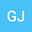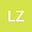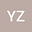Lower Bound of Decay Rate for Higher Order Derivatives of Solution to the Compressible Quantum Magnetohydrodynamic Model
•••• Gao Jincheng,
• Lyu Zeyu,
• Yao Zheng-an
Gao Jincheng
Sun Yat-Sen University
Author ProfileLyu Zeyu
Sun Yat-Sen University
Author ProfileYao Zheng-an
Sun Yat-Sen University
Author Profile## Abstract

The lower bound decay rate of global solution to the compressible viscous quantum magnetohydrodynamic model in three-dimensional whole space under the $H^5\times H^4\times H^4$ framework is investigated in this paper. We firstly show that the lower bound of decay rate for the density, velocity and magnetic field converging to the equilibrium state (1,0,0) in $L^2$-norm is $(1+t)^{-\frac{3}{4}}$ when the initial data satisfies some low frequency assumption. Moreover, we prove that the lower bound of decay rate of $k(k\in [1,3])$ order spatial derivative for the density, velocity and magnetic field converging to the equilibrium state (1,0,0) in $L^2$-norm is $(1+t)^{-\frac{3+2k}{4}}$. Then we show that the lower bound of decay rate for the time derivatives of density and velocity converging to zero in $L^2$-norm is $(1+t)^{-\frac{5}{4}}$, but the lower bound of decay rate for the time derivative of magnetic field converging to zero in $L^2$-norm is $(1+t)^{-\frac{7}{4}}$.
05 Mar 2020Submitted to Mathematical Methods in the Applied Sciences
07 Mar 2020Assigned to Editor
07 Mar 2020Submission Checks Completed
27 Mar 2020Reviewer(s) Assigned
03 Jul 2020Review(s) Completed, Editorial Evaluation Pending
26 Sep 2020Editorial Decision: Accept